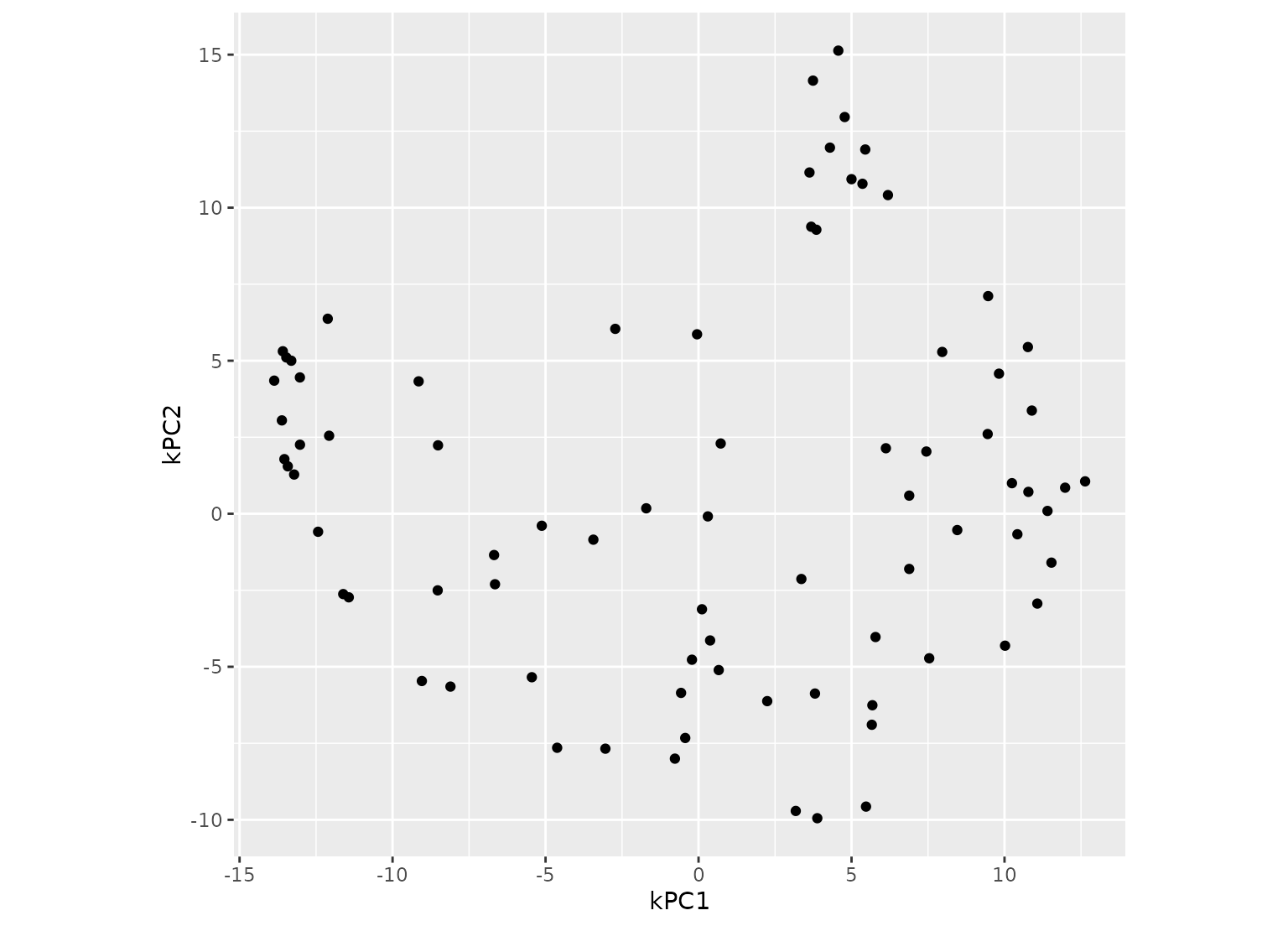step_kpca creates a specification of a recipe step that will convert numeric data into one or more principal components using a kernel basis expansion.

step_kpca(
recipe,
...,
role = "predictor",
trained = FALSE,
num_comp = 5,
res = NULL,
options = list(kernel = "rbfdot", kpar = list(sigma = 0.2)),
prefix = "kPC",
keep_original_cols = FALSE,
skip = FALSE,
id = rand_id("kpca")
)

## Arguments

recipe A recipe object. The step will be added to the sequence of operations for this recipe. One or more selector functions to choose variables for this step. See selections() for more details. For model terms created by this step, what analysis role should they be assigned? By default, the new columns created by this step from the original variables will be used as predictors in a model. A logical to indicate if the quantities for preprocessing have been estimated. The number of PCA components to retain as new predictors. If num_comp is greater than the number of columns or the number of possible components, a smaller value will be used. An S4 kernlab::kpca() object is stored here once this preprocessing step has be trained by prep(). A list of options to kernlab::kpca(). Defaults are set for the arguments kernel and kpar but others can be passed in. Note that the arguments x and features should not be passed here (or at all). A character string for the prefix of the resulting new variables. See notes below. A logical to keep the original variables in the output. Defaults to FALSE. A logical. Should the step be skipped when the recipe is baked by bake.recipe()? While all operations are baked when prep.recipe() is run, some operations may not be able to be conducted on new data (e.g. processing the outcome variable(s)). Care should be taken when using skip = TRUE as it may affect the computations for subsequent operations. A character string that is unique to this step to identify it.

## Value

An updated version of recipe with the new step added to the sequence of any existing operations.

## Details

When performing kPCA with step_kpca(), you must choose the kernel function (and any important kernel parameters). This step uses the kernlab package; the reference below discusses the types of kernels available and their parameter(s). These specifications can be made in the kernel and kpar slots of the options argument to step_kpca(). Consider using step_kpca_rbf() for a radial basis function kernel or step_kpca_poly() for a polynomial kernel.

Kernel principal component analysis (kPCA) is an extension of a PCA analysis that conducts the calculations in a broader dimensionality defined by a kernel function. For example, if a quadratic kernel function were used, each variable would be represented by its original values as well as its square. This nonlinear mapping is used during the PCA analysis and can potentially help find better representations of the original data.

This step requires the dimRed and kernlab packages. If not installed, the step will stop with a prompt about installing these packages.

As with ordinary PCA, it is important to center and scale the variables prior to computing PCA components (step_normalize() can be used for this purpose).

The argument num_comp controls the number of components that will be retained; the original variables that are used to derive the components are removed from the data when keep_original_cols = FALSE. The new components will have names that begin with prefix and a sequence of numbers. The variable names are padded with zeros. For example, if num_comp < 10, the new names will be kPC1 - kPC9. If num_comp = 101, the names would be kPC001 - kPC101.

When you tidy() this step, a tibble with column terms (the selectors or variables selected) is returned.

Scholkopf, B., Smola, A., and Muller, K. (1997). Kernel principal component analysis. Lecture Notes in Computer Science, 1327, 583-588.

Karatzoglou, K., Smola, A., Hornik, K., and Zeileis, A. (2004). kernlab - An S4 package for kernel methods in R. Journal of Statistical Software, 11(1), 1-20.

Other multivariate transformation steps: step_classdist(), step_depth(), step_geodist(), step_ica(), step_isomap(), step_kpca_poly(), step_kpca_rbf(), step_mutate_at(), step_nnmf(), step_pca(), step_pls(), step_ratio(), step_spatialsign()

## Examples

library(modeldata)
data(biomass)

biomass_tr <- biomass[biomass$dataset == "Training",] biomass_te <- biomass[biomass$dataset == "Testing",]

rec <- recipe(HHV ~ carbon + hydrogen + oxygen + nitrogen + sulfur,
data = biomass_tr)

kpca_trans <- rec %>%
step_YeoJohnson(all_numeric_predictors()) %>%
step_normalize(all_numeric_predictors()) %>%
step_kpca(all_numeric_predictors())

if (require(dimRed) & require(kernlab)) {
kpca_estimates <- prep(kpca_trans, training = biomass_tr)

kpca_te <- bake(kpca_estimates, biomass_te)

rng <- extendrange(c(kpca_te$kPC1, kpca_te$kPC2))
plot(kpca_te$kPC1, kpca_te$kPC2,
xlim = rng, ylim = rng)

tidy(kpca_trans, number = 3)
tidy(kpca_estimates, number = 3)
}#> # A tibble: 5 × 2
#>   terms    id
#>   <chr>    <chr>
#> 1 carbon   kpca_Qw9rI
#> 2 hydrogen kpca_Qw9rI
#> 3 oxygen   kpca_Qw9rI
#> 4 nitrogen kpca_Qw9rI
#> 5 sulfur   kpca_Qw9rI